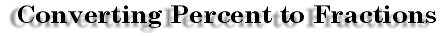Percent to Fraction Converting Percent to Fractions

###Recall that percent literally means "out of 100." If you are given 45%, you know that this translates into 45 out of 100 or 45 / 100. This fraction can then be reduced to 9 / 20.

 When given percents containing decimals such as 25.5%, follow a similar procedure. Change 25.5% to 25.5 / 100. Now, move the decimal place to the right in the numerator and the denominator until you have rid the fraction of decimals. Hence 25.5 / 100 becomes 255 / 1000. Reduced 255 / 1000 = 51 / 200. Sometimes,the percents are less than 1… .7% = .7 / 100 = 7 / 1000Sometimes the percents are greater than 100… 125% = 125 / 100 = 1 25/100 = 1 1/4 In this case, we turned the percent into an improper fraction, then to a mixed number, then we reduced.If 15% of a fabric store's yarn is blue, what will the numerator be if the denominator is 400?

Calculating the Percent of Numbers

 writer:Kevin Gallagher

Percent